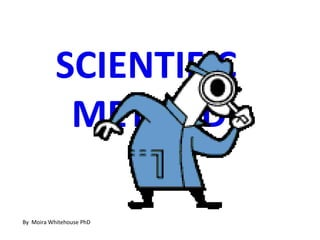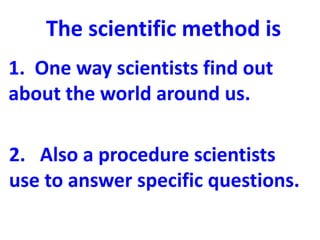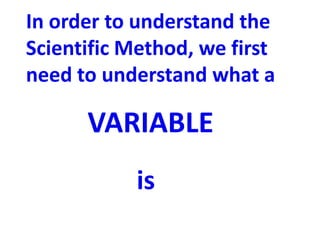Successfully reported this slideshow.

# Scientific Method Variables (Teach)

64

Share×
1 of 45
1 of 45

# Scientific Method Variables (Teach)

64

Share

Elementary introduction to the scientific method focusing on variables. This is the first of a two part lesson on the scientific method which focuses variables and the later one covers the procedures of the scientific method, at about the 5th and 6th grade level.
The second slide show is called Scientific Method Procedures.

Elementary introduction to the scientific method focusing on variables. This is the first of a two part lesson on the scientific method which focuses variables and the later one covers the procedures of the scientific method, at about the 5th and 6th grade level.
The second slide show is called Scientific Method Procedures.

## More Related Content

### Related Books

Free with a 14 day trial from Scribd

See all

### Related Audiobooks

Free with a 14 day trial from Scribd

See all

### Scientific Method Variables (Teach)

1. 1. SCIENTIFIC METHOD Complemented by my slideshow Scientific Method Procedures By Moira Whitehouse PhD
2. 2. The scientific method is 1. One way scientists find out about the world around us. 2. Also a procedure scientists use to answer specific questions.
3. 3. In order to understand the Scientific Method, we first need to understand what a VARIABLE is
4. 4. A variable is anything in an experiment that can change.
5. 5. Here you see a car sitting on top of a ramp. You want to design a car that will roll down a ramp really fast. So you need to know what things affect how fast a car will roll down a ramp. What could you change about this car or ramp that might affect its speed?
6. 6. Yes, what could you change or do to make the car go faster. These ideas all make up something we call:
7. 7. variables
8. 8. There are three main kinds of variables 1. The change variable 2. The responding variable 3. The control variables
9. 9. Let’s look more closely at these three kinds of variables Remember the first is called the change variable.
10. 10. 1. The change variable is: • There can only be one change variable in any experiment. • The variable that the experimenter changes on purpose. • May also be called the independent variable. (two names for the same variable)
11. 11. In the experiment on the next page, identify the change variable—what is the one thing the experimenter changed with plant 1, 2 and 3?
12. 12. Water Water every every day day No Water 20 mL 40 mL Plant 1 Plant 2 Plant 3
13. 13. Yes, the experiment changed the amount of water that the plant receives each day.
14. 14. The second type of variable is 2. The responding variable
15. 15. 2. The responding variable is • What is measured or observed by the experimenter to see if the change variable caused a different result.
16. 16. The responding variable is sometimes called the dependent variable. (again, two names for the same variable) How this variable responds (goes faster, grows taller, warms up more, etc.) depends on the change (or independent) variable.
17. 17. Let’s look again at the experiment where the amount of water each plant received was changed. What could the experimenter observe or measure to see if the amount of water made a difference to the health of the plant?
18. 18. Water Water every every day day No Water 20 mL 40 mL Plant 1 Plant 2 Plant 3
19. 19. The experimenter could have, for example, counted the number of green leaves on each plant or he could have measured the height of the plants after 14 days.
20. 20. Some examples of responding variables in different experiments—things to be observed or measured are: The amount of water absorbed by two different brands of paper towels. How far a ball rolls from different ramp angles. The amount of feed eaten at a bird feeder in response to the type of seed in the feeder.
21. 21. Whenever possible the experimenter should measure, instead of just observing, the responding variable. In order to measure the responding variable the experimenter uses tools such as
22. 22. a metric ruler or meter stick To measure how far something moved.
23. 23. balance scale to measure the mass of something
24. 24. thermometer To measure how hot or cold something is
25. 25. What might the change variable be in this experiment on water evaporating? without fan with fan that moves the air What could be the responding variable in this experiment? Remember you should measure.
26. 26. PLASTIC STYROFOAM CUP OF CUP OF HOT HOT WATER WATER What might the change variable be in this experiment on keeping water hot? What might be the responding variable in this experiment? Remember you should measure.
27. 27. What would the What could be the change variable responding be in this variable in this experiment on experiment? how much heat Remember you different soils should measure. absorb?
28. 28. To use the scientific method to answer questions, we set up an experiment that changes just one thing and then we measure how that one change affects what we are interested in. The question is always “what is the effect of change variable on responding variable”?
29. 29. For example, in the case of our watering plants experiment, the question might be: How does the amount of water you give a plant (change variable) affect how tall the plant grows. (responding variable)?
30. 30. But there is more. In every experiment there is a third kind of variable called the control variable. Control variables are all those things in an experiment that you do not let change.
31. 31. • A control variable is any other variable (other than the change variable) that might affect the outcome of the experiment. • There may be many control variables in any experiment • Control variables must be kept constant between trials.
32. 32. Think back to our experiment asking the question: “How does the amount of water affect how tall the plant grows in 14 days?” What variables, other than the amount of water, could affect how tall the plant grows?
33. 33. Water Water every every day day No Water 20 mL 40 mL Plant 1 Plant 2 Plant 3
34. 34. Things that might affect how tall the plant grows could include: 1. the amount of light 2. the temperature of the air 3. the kind of soil
35. 35. What would be the problem if we changed both the amount of water and the type of soil the plant is growing in at the same time? Sure, you wouldn’t know if the growth was due to the water or the type of soil.
36. 36. Water Water every every day day No Water Potting soil Sandy soil Clay soil 20 mL 40 mL Plant 1 Plant 2 Plant 3
37. 37. Suppose the plant in the potting soil that was given 40 mL of water daily grew the tallest after 14 days? Could we know whether the 40 mL of water or planting the plant in potting soil had caused the results?
38. 38. Probably not: In order to make correct conclusions when doing experiments we must: • have only one change variable and • control all other variables that might affect the results— keep them constant through out the experiment.
39. 39. Yes, you would have to plant all plants in the same potting soil, use the same kind of pot, expose all plants to the same amount of light and keep the air temperature the same.
40. 40. So let’s look at that toy car on the ramp again. Now, you want to find out if making the angle of the ramp higher would make the car coast further. So your question is: Will increasing the angle of the ramp make my car roll further?
41. 41. •What would your change variable be? •What would your responding variable be? •What would some of your control variables be?
42. 42. Control variables?. What’s wrong with this picture? MADE OF PLASTIC MADE OF WOOD